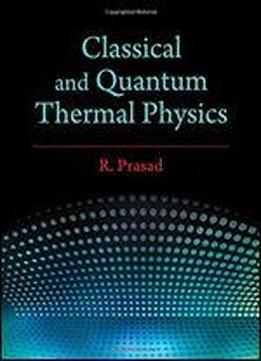# Classical And Quantum Thermal Physics by R. Prasad / 2016 / English / PDF

Covering essential areas of thermal physics, this book includes kinetic theory, classical thermodynamics, and quantum thermodynamics. The text begins by explaining fundamental concepts of the kinetic theory of gases, viscosity, conductivity, diffusion, and the laws of thermodynamics and their applications. It then goes on to discuss applications of thermodynamics to problems of physics and engineering. These applications are explained with the help of P-V and P-S-H diagrams where necessary and are followed by a large number of solved examples and unsolved exercises. The book includes a dedicated chapter on the applications of thermodynamics to chemical reactions. Each application is explained by taking the example of an appropriate chemical reaction, where all technical terms are explained and complete mathematical derivations are worked out in steps starting from the first principle.

views: 247